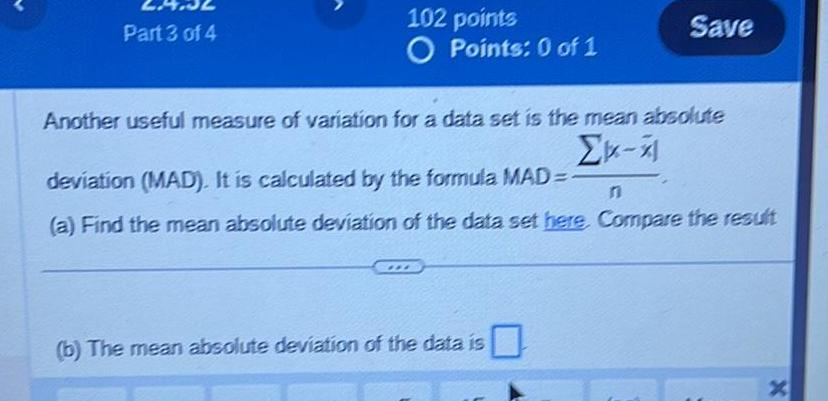Question:

# Part 3 of 4 102 points O Points 0 of 1 Save Another useful

Last updated: 9/8/2023Part 3 of 4 102 points O Points 0 of 1 Save Another useful measure of variation for a data set is the mean absolute X deviation MAD It is calculated by the formula MAD a Find the mean absolute deviation of the data set here Compare the result b The mean absolute deviation of the data is X